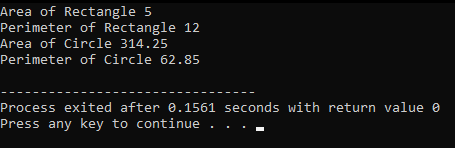# Abstract Classes in C++

## Abstract Classes in C++ with Examples:

In this article, I am going to discuss Abstract Classes in C++ with Examples. Please read our previous article where we discussed Runtime Polymorphism in C++ with Example.

##### What is Abstract Class in C++?

An abstract class in C++ has at least one pure virtual function by definition. In other words, a function that has no definition and these classes cannot be instantiated. The abstract class’s child classes must provide body to the pure virtual function; otherwise, the child class would become an abstract class in its own right.

##### Example to Understand Abstract Classes in C++:

Before understanding abstract classes, let me give you a complete idea about the inheritance and reusability, and then we will discuss the abstract classes in C++. For this, we will take a simple example and discuss everything. Suppose we have a class called Base as shown in the below image.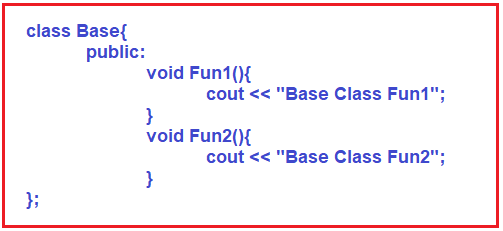This is our Base class. This class is having 2 methods that are Fun1 and Fun2. These 2 functions will display 2 different messages on the screen. Next, we have another class that is Derived class as shown in the below image. Here, the Derived class is inherited from the Base class.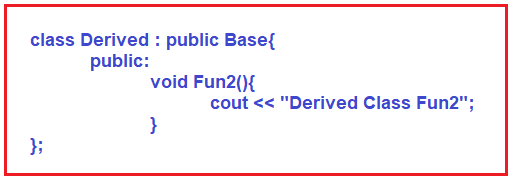This is the example where a Derived class is reusing the functions of the Base class. Because the Derived class is inherited from the Base class, so everything present in the Base class is also available in the Derived class. So, it’s borrowing the features. Base class sharing its features with the derived classes. This is reusability. So, one purpose of inheritance is reusability.

Next, we have written Fun2 inside the Derived class. Here we have overridden Fun2. Redefining a function of a base class in a child class that is a derived class is called function overriding. It is redefining the function. Now, the question is, when it is reusing or borrowing then what is the need of redefining it?

When you are saying you want to redefine then it means you want to achieve polymorphism. So, the second purpose of inheritance is polymorphism. Do you think when a Derived class is having Fun2 function then the base class should have the body of the function? In some cases, yes, we need so we keep it. In some cases, no, only for the namesake it is having. Let the derived class have the overridden body of that function.

It means we want to achieve polymorphism. So, for achieving polymorphism we need the functions in the base class as virtual. Saying that they are not real. They are just for namesake and the real function is in the child class which is overridden.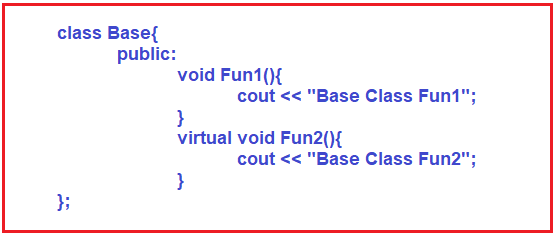Already we have discussed that by using a base class pointer how we can access the overridden functions.

Now the next thing, when you have Fun2 as a virtual function in Base class, do you think we need the body? No, we don’t need the body. We want the body of that function only in the derived classes. The Base class has no functionality at all. It is just having a function. Just a prototype of a function. So let us remove the body of fun2 in class Base as shown in the below image.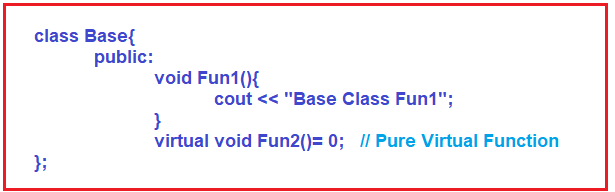If we just removed the body that will not do. You have to implement the functions so either you have to do it outside using scope resolution. We also don’t want to do that. So, for that, we have assigned Fun2 to zero. This is called a pure virtual function.

##### What is the purpose of the Pure Virtual function?

The purpose of a pure virtual function is to achieve polymorphism. We want the derived classes to override this function. So, it becomes mandatory for derived classes to override the pure virtual function. It means the base class is governing or giving a rule to the child classes that you must override the pure virtual functions. So, it is defining an interface.

Pure virtual functions are useful for defining an interface. Now final statement, if a class is having a pure virtual function, then that class is called an Abstract Class in C++. Can we create the object of Abstract Class in C++? No. Can we create the pointer of the abstract class? Yes. For a better understanding, please have a look at the below image. Here, Base is an abstract class as it contains one pure virtual function i.e. Fun2.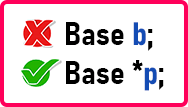So abstract classes in C++ cannot be instantiated. We cannot have the objects but we can have a pointer to achieve polymorphism. How to achieve polymorphism? Already we have shown you in our previous articles that you can assign the object of the derived class to the base class pointer as follows:
Base *p = new Derived();

Then we can call functions present in the Base class as follows:
p->Fun2();

But whose function will be called here? Derived class Fun2 will be called. This we have already seen. This is for achieving polymorphism. So, this is all about abstract classes in C++.

##### Now the conclusion, what is the purpose of inheritance?

Two things, one is reusability. The derived class gets the function from the base class. The second thing to achieve is polymorphism. What is that? There is a pure virtual function in the base class so the derived class must override that function. So that’s it.

##### Types of Classes in C++:

There are three things or three types of classes that we can write. Let us see.

1. The first is a base class with all concrete functions. What is the purpose of this? Reusability.
2. Second, if a base class is having some concrete functions and some pure virtual functions then the purpose is reusability as well as polymorphism. This class is known as abstract class.
3. Third, if a base class is having all pure virtual functions, then the only purpose is polymorphism. This class is also known as an abstract class or we can say interface.

For a better understanding, please have a look at the below image.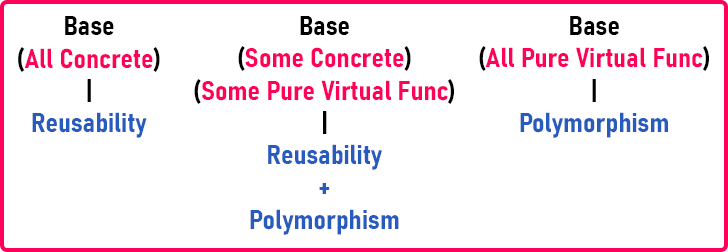So, in C++ we can have these three types of classes. The purpose of the class we have shown above. The interface is not a keyword in C++ just we are calling it an interface. This is a category name we’re giving. So, it’s an abstract class.

But when it comes to Java or C#, such classes are called interface only and there is a separate keyword called the interface. There is something different than classes but C++ doesn’t have that. For interfaces also write the class in C++. That’s why C++ supports multiple inheritances.

##### Key Points of Abstract class in C++:
1. The class having a pure virtual function is an abstract class
2. An abstract class can have concrete also.
3. The object of abstract class cannot be created
4. A derived class must override pure virtual function, otherwise, it will also become an abstract class.
5. The pointer of the abstract class can be created
6. The pointer of the abstract class can hold the object of the derived class
7. Abstract classes are used for achieving polymorphism
8. The base class can be concrete
9. Abstract with some concrete and some pure virtual functions

That it. So, we have explained to you about abstract classes. Now let us look at the program.

##### Program to understand Abstract Classes in C++:
```#include <iostream>
using namespace std;

class Base
{
public:
void Fun1()
{
cout << "Base Class Fun1"<<endl;
}
virtual void Fun2() = 0;	// Pure Virtual Function
};

class Derived:public Base
{
public:
void Fun2()
{
cout << "Derived Class Fun2"<<endl;
}
};

int main()
{
Derived d;
d.Fun1();
d.Fun2();
return 0;
}
```
###### Output: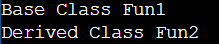##### Realtime Example to Understand Abstract Classes in C++:
```#include <iostream>
using namespace std;

class Shape
{
public:
virtual float Area() = 0;
virtual float Perimeter() = 0;
};

class Rectangle:public Shape
{
private:
float length;

public:
Rectangle(int l = 1, int b = 1)
{
length = 1;
}
float Area()
{
}
float Perimeter()
{
return 2 * (length + breadth);
}
};

class Circle:public Shape
{
private:
public:
Circle(float r)
{
}
float Area()
{
}
float Perimeter()
{
return 2 * 3.1425 * radius;
}
};

int main()
{
Shape *s = new Rectangle(10, 5);
cout << "Area of Rectangle " << s->Area() << endl;
cout << "Perimeter of Rectangle " << s->Perimeter() << endl;

s = new Circle(10);
cout << "Area of Circle " << s->Area() << endl;
cout << "Perimeter of Circle " << s->Perimeter() << endl;
}
```
###### Output: